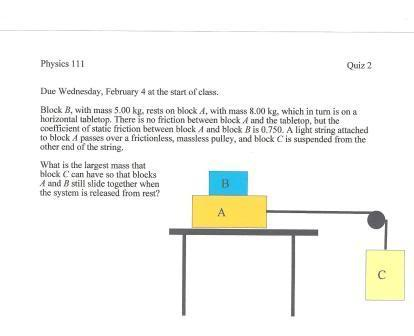# Blocks on a tabletop attached to a mass suspended by a pulley

## Homework Statement## Homework Equations

We have yet to go over this material, which kinda pisses me off, but I'm guessing that:

f=ma, w=ma, g = 9.8m/s/s

are important

## The Attempt at a Solution

If somebody could point me in the right direction that would be very helpful. I attempted at drawing a diagram of the forces on each object, but I'm not sure where to even start.

## Answers and Replies

You want to find the mass of block C so that it moves block A but not too large that it overcomes the static friction between block A and block B I believe. So find the static friction and divide it by g to find the mass of Block C

static friction = (coefficient of static friction) (reaction/normal force)

So will the normal force be the mass of the two blocks times gravity?

the normal force should be equal to the weight of Block B

Thank you so much

I got 3.75 kg